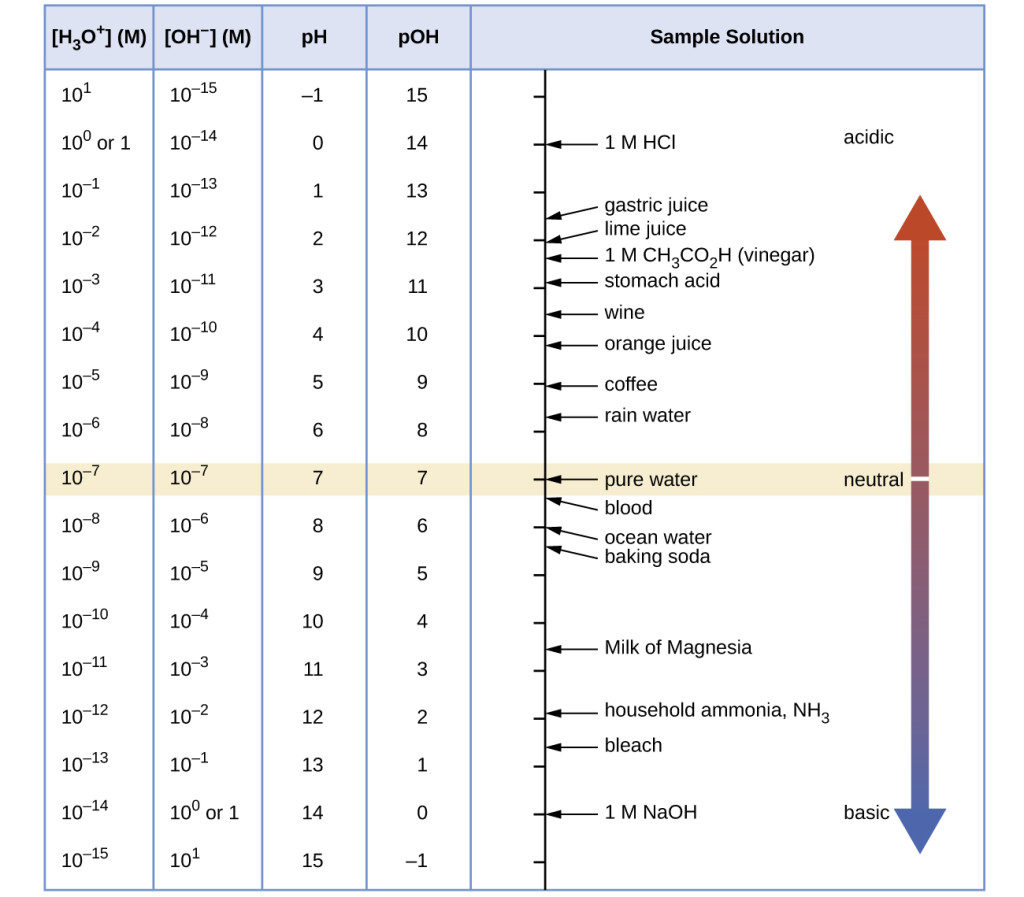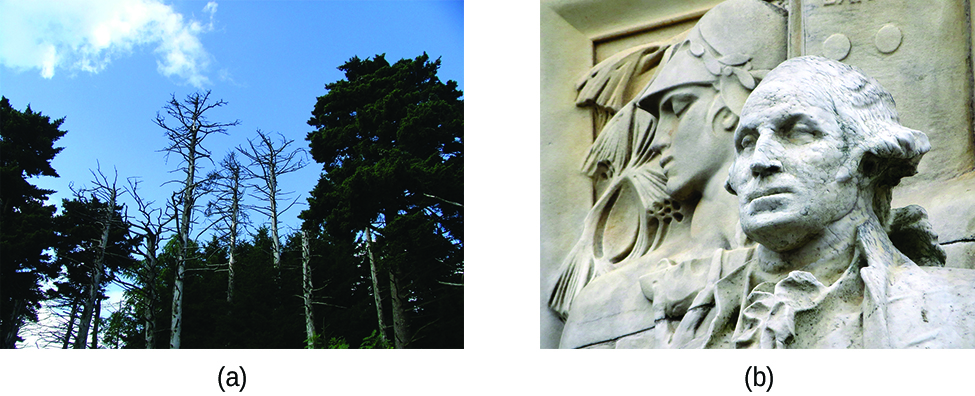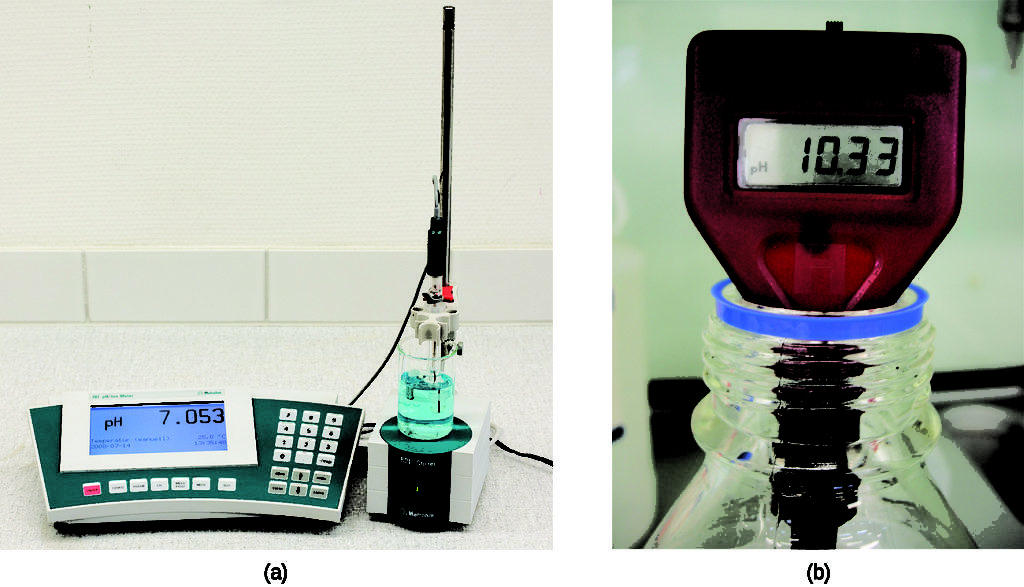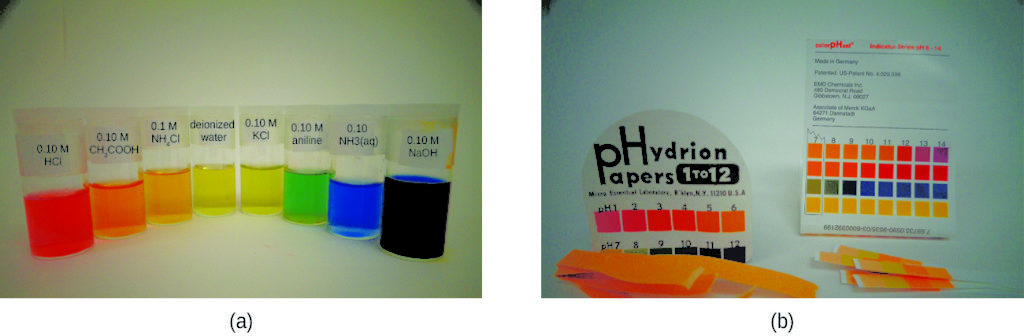## pH and pOH

### Learning Objectives

By the end of this module, you will be able to:

• Explain the characterization of aqueous solutions as acidic, basic, or neutral
• Express hydronium and hydroxide ion concentrations on the pH and pOH scales
• Perform calculations relating pH and pOH

As discussed earlier, hydronium and hydroxide ions are present both in pure water and in all aqueous solutions, and their concentrations are inversely proportional as determined by the ion product of water (Kw). The concentrations of these ions in a solution are often critical determinants of the solution’s properties and the chemical behaviors of its other solutes, and specific vocabulary has been developed to describe these concentrations in relative terms. A solution is neutral if it contains equal concentrations of hydronium and hydroxide ions; acidic if it contains a greater concentration of hydronium ions than hydroxide ions; and basic if it contains a lesser concentration of hydronium ions than hydroxide ions.

A common means of expressing quantities, the values of which may span many orders of magnitude, is to use a logarithmic scale. One such scale that is very popular for chemical concentrations and equilibrium constants is based on the p-function, defined as shown where “X” is the quantity of interest and “log” is the base-10 logarithm:

pX = −log X

The pH of a solution is therefore defined as shown here, where [H3O+] is the molar concentration of hydronium ion in the solution:

pH = −log[H3O+]

Rearranging this equation to isolate the hydronium ion molarity yields the equivalent expression:

[H3O+] = 10−pH

Likewise, the hydroxide ion molarity may be expressed as a p-function, or pOH:

pOH = −log[OH]

or

[OH] = 10−pOH

Finally, the relation between these two ion concentration expressed as p-functions is easily derived from the Kw expression:

K= [H3O+][OH]
−logKw = −log([H3O+][OH]) = −log[H3O+] + −log[OH]
pK= pH + pOH

At 25 °C, the value of Kw is 1.0 × 10−14, and so:

14.00 = pH +pOH

As we learned earlier, the hydronium ion molarity in pure water (or any neutral solution) is 1.0 × 10−7 M at 25 °C. The pH and pOH of a neutral solution at this temperature are therefore:

pH = −log[H3O+] = −log(1.0 × 10−7) = 7.00

pOH = −log[OH] = −log(1.0 × 10−7) = 7.00

And so, at this temperature, acidic solutions are those with hydronium ion molarities greater than 1.0 × 10−7 M and hydroxide ion molarities less than 1.0 × 10−7 M (corresponding to pH values less than 7.00 and pOH values greater than 7.00). Basic solutions are those with hydronium ion molarities less than 1.0 × 10−7 M and hydroxide ion molarities greater than 1.0 × 10−7 M (corresponding to pH values greater than 7.00 and pOH values less than 7.00).

Since the autoionization constant Kw is temperature dependent, these correlations between pH values and the acidic/neutral/basic adjectives will be different at temperatures other than 25 °C. For example, the hydronium molarity of pure water is 80 °C is 4.9 × 10−7 M, which corresponds to pH and pOH values of:

pH = −log[H3O+] = −log(4.9 × 10−7) = 6.31

pOH = −log[OH] = −log(4.9 × 10−7) = 6.31

At this temperature, then, neutral solutions exhibit pH = pOH = 6.31, acidic solutions exhibit pH less than 6.31 and pOH greater than 6.31, whereas basic solutions exhibit pH greater than 6.31 and pOH less than 6.31. This distinction can be important when studying certain processes that occur at nonstandard temperatures, such as enzyme reactions in warm-blooded organisms. Unless otherwise noted, references to pH values are presumed to be those at standard temperature (25 °C) (Table 1).

Table 1. Summary of Relations for Acidic, Basic and Neutral Solutions
Classification  Relative Ion Concentrations  pH at 25 °C
acidic  [H3O+] > [OH]  pH < 7
neutral  [H3O+] = [OH]  pH = 7
basic  [H3O+] < [OH]  pH > 7

Figure 1 shows the relationships between [H3O+], [OH], pH, and pOH, and gives values for these properties at standard temperatures for some common substances.Figure 1. The pH and pOH scales represent concentrations of [H3O+] and OH, respectively. The pH and pOH values of some common substances at standard temperature (25 °C) are shown in this chart.

### Example 1: Calculation of pH from [H3O+]

What is the pH of stomach acid, a solution of HCl with a hydronium ion concentration of 1.2 × 10–3 M?

Water exposed to air contains carbonic acid, H2CO3, due to the reaction between carbon dioxide and water:

CO2(aq) + H2O(l) ⇌ H2CO3(aq)

Air-saturated water has a hydronium ion concentration caused by the dissolved CO2 of 2.0 × 10–6 M, about 20-times larger than that of pure water. Calculate the pH of the solution at 25 °C.

### Example 2: Calculation of Hydronium Ion Concentration from pH

Calculate the hydronium ion concentration of blood, the pH of which is 7.3 (slightly alkaline).

Calculate the hydronium ion concentration of a solution with a pH of −1.07.

### Environmental Science

Normal rainwater has a pH between 5 and 6 due to the presence of dissolved CO2 which forms carbonic acid:

H2O(l)+ CO2(g) ⟶ H2CO3(aq)

H2CO3(aq) ⇌ H+(aq)+HCO3(aq)

Acid rain is rainwater that has a pH of less than 5, due to a variety of nonmetal oxides, including CO2, SO2, SO3, NO, and NO2 being dissolved in the water and reacting with it to form not only carbonic acid, but sulfuric acid and nitric acid. The formation and subsequent ionization of sulfuric acid are shown here:

H2O(l)+SO3(g) ⟶ H2SO4(aq)

H2SO4(aq) ⟶ H+(aq) + HSO2(aq)

Carbon dioxide is naturally present in the atmosphere because we and most other organisms produce it as a waste product of metabolism. Carbon dioxide is also formed when fires release carbon stored in vegetation or when we burn wood or fossil fuels. Sulfur trioxide in the atmosphere is naturally produced by volcanic activity, but it also stems from burning fossil fuels, which have traces of sulfur, and from the process of “roasting” ores of metal sulfides in metal-refining processes. Oxides of nitrogen are formed in internal combustion engines where the high temperatures make it possible for the nitrogen and oxygen in air to chemically combine.

Acid rain is a particular problem in industrial areas where the products of combustion and smelting are released into the air without being stripped of sulfur and nitrogen oxides. In North America and Europe until the 1980s, it was responsible for the destruction of forests and freshwater lakes, when the acidity of the rain actually killed trees, damaged soil, and made lakes uninhabitable for all but the most acid-tolerant species. Acid rain also corrodes statuary and building facades that are made of marble and limestone (Figure 2). Regulations limiting the amount of sulfur and nitrogen oxides that can be released into the atmosphere by industry and automobiles have reduced the severity of acid damage to both natural and manmade environments in North America and Europe. It is now a growing problem in industrial areas of China and India.

For further information on acid rain, visit this US Environmental Protection Agency website.Figure 2. (a) Acid rain makes trees more susceptible to drought and insect infestation, and depletes nutrients in the soil. (b) It also is corrodes statues that are carved from marble or limestone. (credit a: modification of work by Chris M Morris; credit b: modification of work by “Eden, Janine and Jim”/Flickr)

### Example 3: Calculation of pOH

What are the pOH and the pH of a 0.0125-M solution of potassium hydroxide, KOH?

The hydronium ion concentration of vinegar is approximately 4 × 10–3 M. What are the corresponding values of pOH and pH?

The acidity of a solution is typically assessed experimentally by measurement of its pH. The pOH of a solution is not usually measured, as it is easily calculated from an experimentally determined pH value. The pH of a solution can be directly measured using a pH meter (Figure 3).Figure 3. (a) A research-grade pH meter used in a laboratory can have a resolution of 0.001 pH units, an accuracy of ± 0.002 pH units, and may cost in excess of \$1000. (b) A portable pH meter has lower resolution (0.01 pH units), lower accuracy (± 0.2 pH units), and a far lower price tag. (credit b: modification of work by Jacopo Werther)

The pH of a solution may also be visually estimated using colored indicators (Figure 4).Figure 4. (a) A universal indicator assumes a different color in solutions of different pH values. Thus, it can be added to a solution to determine the pH of the solution. The eight vials each contain a universal indicator and 0.1-M solutions of progressively weaker acids: HCl (pH = l), CH3CO2H (pH = 3), and NH4Cl (pH = 5), deionized water, a neutral substance (pH = 7); and 0.1-M solutions of the progressively stronger bases: KCl (pH = 7), aniline, C6H5NH2 (pH = 9), NH3 (pH = 11), and NaOH (pH = 13). (b) pH paper contains a mixture of indicators that give different colors in solutions of differing pH values. (credit: modification of work by Sahar Atwa)

### Key Concepts and Summary

The concentration of hydronium ion in a solution of an acid in water is greater than 1.0 × 10−7 M at 25 °C. The concentration of hydroxide ion in a solution of a base in water is greater than 1.0 × 10−7 M at 25 °C. The concentration of H3O+ in a solution can be expressed as the pH of the solution; pH = −logH3O+. The concentration of OH can be expressed as the pOH of the solution: pOH = −log[OH]. In pure water, pH = 7.00 and pOH = 7.00

#### Key Equations

• pH = −log[H3O+]
• pOH = −log[OH]
• [H3O+] =10−pH
• [OH] =10−pOH
• pH + pOH = pKw = 14.00 at 25 °C

### Exercises

1. Explain why a sample of pure water at 40 °C is neutral even though [H3O+] = 1.7 × 10−7 MKw is 2.9 × 10−14 at 40 °C.
2. The ionization constant for water (Kw) is 2.9 × 10−14 at 40 °C. Calculate [H3O+], [OH], pH, and pOH for pure water at 40 °C.
3. The ionization constant for water (Kw) is 9.614 × 10−14 at 60 °C. Calculate [H3O+], [OH], pH, and pOH for pure water at 60 °C.
4. Calculate the pH and the pOH of each of the following solutions at 25 °C for which the substances ionize completely:
1. 0.200 M HCl
2. 0.0143 M NaOH
3. 3.0 M HNO3
4. 0.0031 Ca(OH)2
5. Calculate the pH and the pOH of each of the following solutions at 25 °C for which the substances ionize completely:
1. 0.000259 M HClO4
2. 0.21 M NaOH
3. 0.000071 M Ba(OH)2
4. 2.5 M KOH
6. What are the pH and pOH of a solution of 2.0 M HCl, which ionizes completely?
7. What are the hydronium and hydroxide ion concentrations in a solution whose pH is 6.52?
8. Calculate the hydrogen ion concentration and the hydroxide ion concentration in wine from its pH. See Figure 1 for useful information.
9. Calculate the hydronium ion concentration and the hydroxide ion concentration in lime juice from its pH. See Figure 1 for useful information.
10. The hydronium ion concentration in a sample of rainwater is found to be 1.7 × 10−6 M at 25 °C. What is the concentration of hydroxide ions in the rainwater?
11. The hydroxide ion concentration in household ammonia is 3.2 × 10−3 M at 25 °C. What is the concentration of hydronium ions in the solution?

## Glossary

acidic: describes a solution in which [H3O+] > [OH]

basic: describes a solution in which [H3O+] < [OH]

neutral: describes a solution in which [H3O+] = [OH]

pH: logarithmic measure of the concentration of hydronium ions in a solution

pOH: logarithmic measure of the concentration of hydroxide ions in a solution